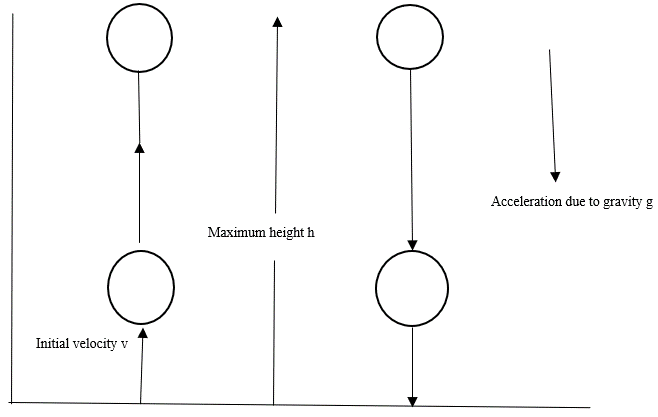A stone thrown vertically upward with 50 m/s velocity and g=10 m/s2. What will be the maximum height achieved by stone, net displacement and total distance covered by stone?

# A stone thrown vertically upward with velocity and . What will be the maximum height achieved by stone, net displacement and total distance covered by stone?

1. A
2. B
3. C
4. D
None of above

Fill Out the Form for Expert Academic Guidance!l

+91

Live ClassesBooksTest SeriesSelf Learning

Verify OTP Code (required)

### Solution:

The maximum height achieved by the stone is net displacement is zero and distance covered by the stone is
Given,
Initial velocity,
Acceleration,
Final velocity, (after achieving maximum height, the stone is at rest)
According to newton’s third equation of motion,
$⇒$
$⇒$
And the stone will fall to its initial position (on earth)
So total displacement will be $0$ and total distance will be twice the maximum height.
Total distance,  .## Related content

 Difference Between Mass and Weight Differences & Comparisons Articles in Physics Important Topic of Physics: Reynolds Number Distance Speed Time Formula Refractive Index Formula Mass Formula Electric Current Formula Electric Power Formula Resistivity Formula Weight Formula+91

Live ClassesBooksTest SeriesSelf Learning

Verify OTP Code (required)